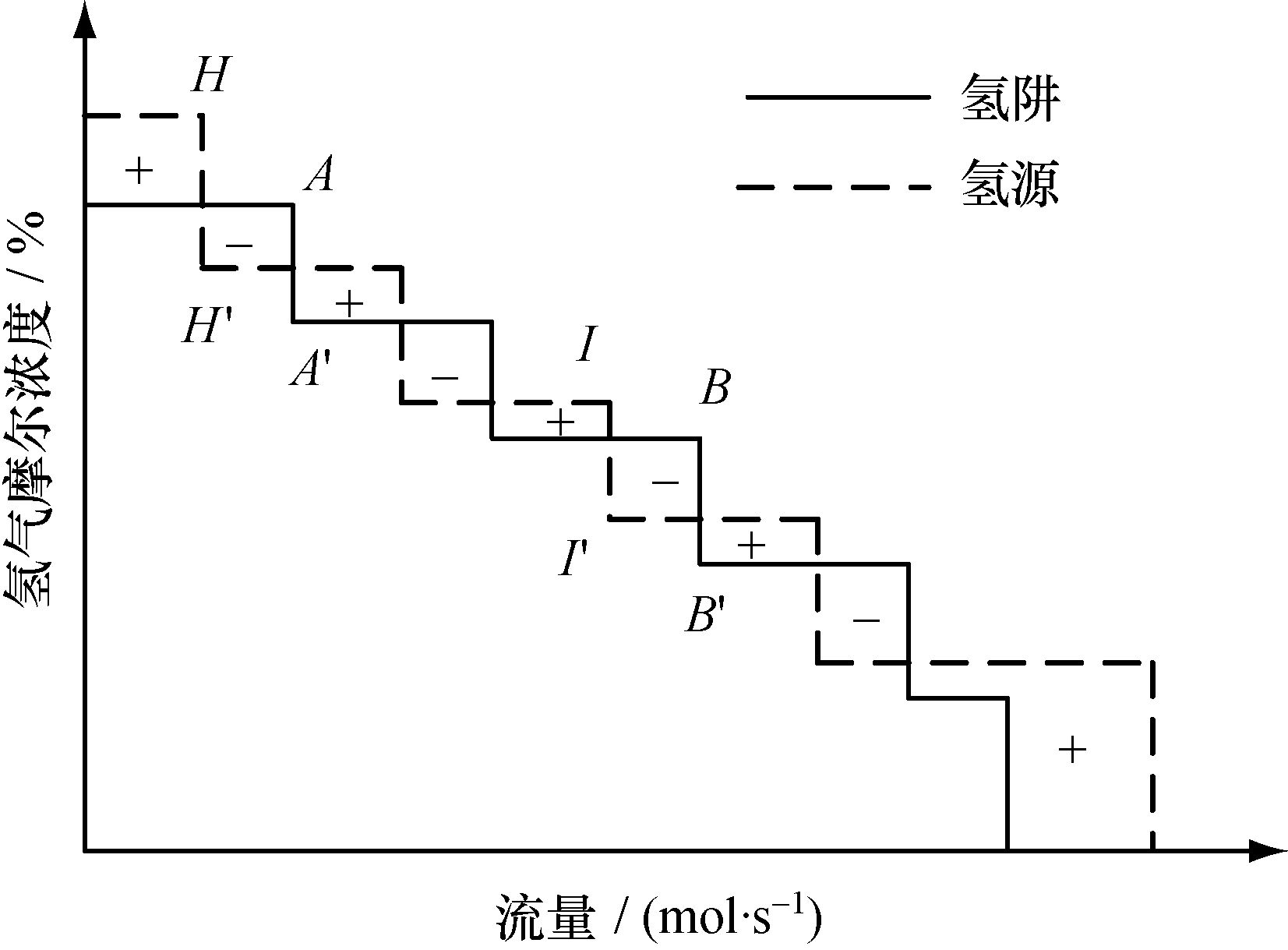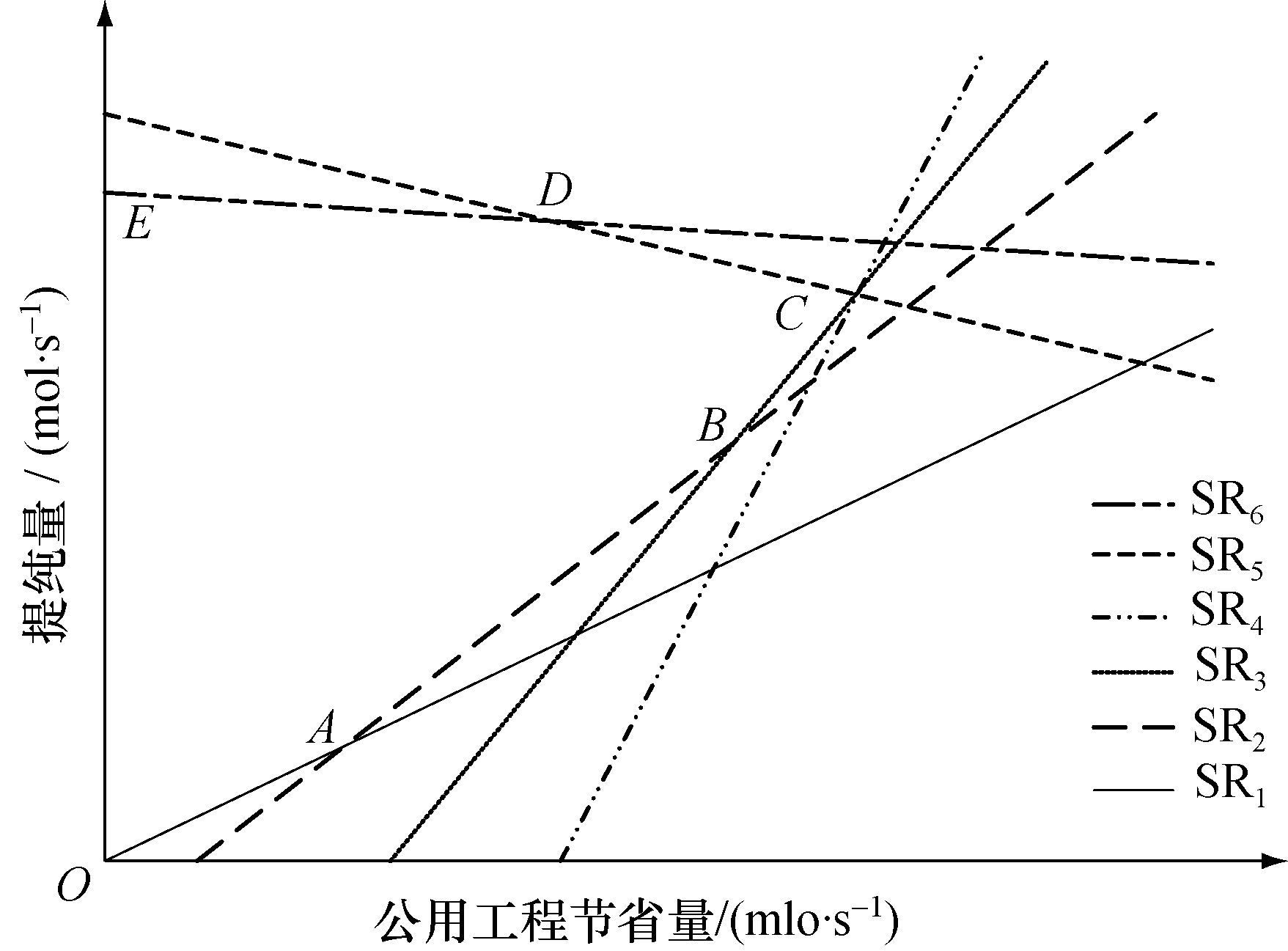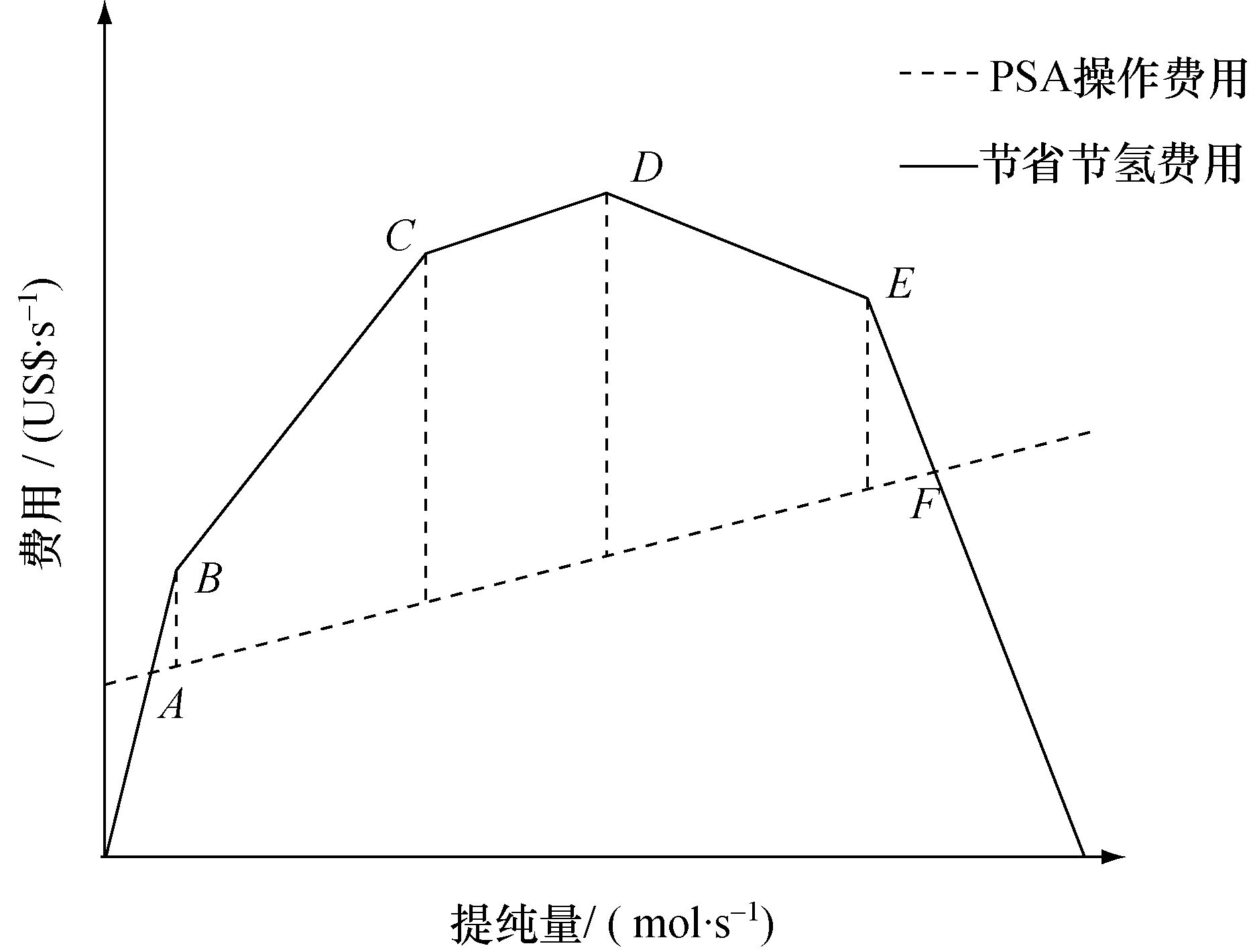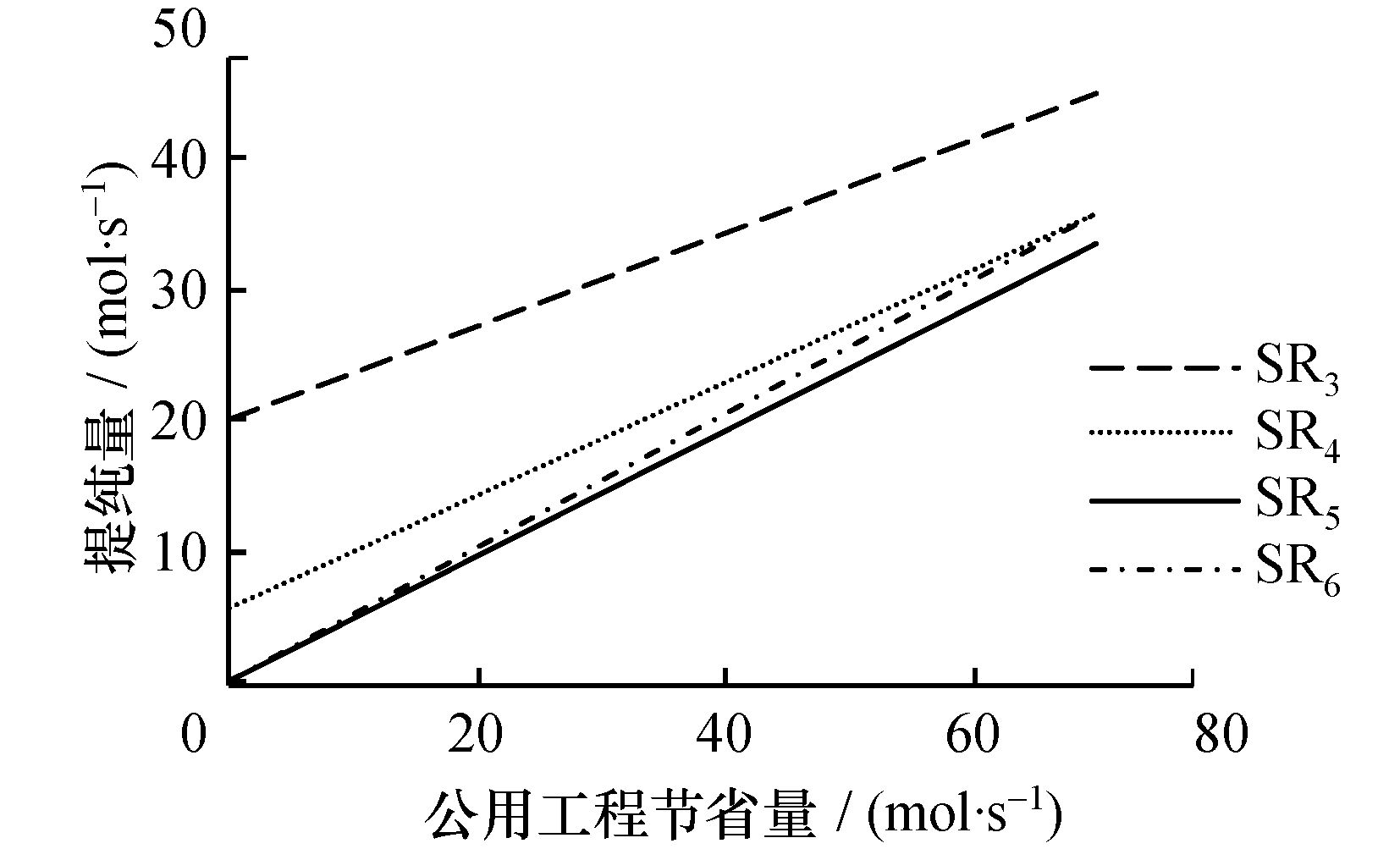Key words: hydrogen network     purification     energy consumption     optimization

Alves等和Liu等通过分析氢气提纯设备，提出3种可能存在的情况(夹点之上、 穿越夹点和夹点之下)，并确定了最优的提纯设置是跨越夹点的提纯。Foo和Manan将性质级联法(PCA)推广至可用于气体网络集成的气体级联法(GCA)，可用于分析提纯回用氢网络。 但这种方法把提纯作为一个固定过程，而未考虑其优化。 Ng等[4-7]将级联分析法扩展为单杂质物质回用网络自动确定目标值的求解方法，并在此基础上提出基于性质和浓度的回用网络优化方法，可确定物质回用网络系统的夹点、 最小公用工程以及最大回用网络，也可用于确定提纯回用氢网络中的最优提纯量。 Nelson和Liu提出了一种易于识别系统夹点和处理多夹点问题的Excel表格法。 该方法虽考虑了提纯回用，但同气体级联法(GCA)一样，未对提纯过程进行优化。 Bandyopadhyay提出的方法同样也可以确定考虑提纯回用氢网络的最小公用工程用量。 基于提纯三角形规则，Zhang等提出一种分析提纯回用氢网络系统的图像法，可在氢负荷-流量图上确定最终的提纯进料量、 提纯产品量、 提纯尾气量以及提纯后系统的夹点位置和最小公用工程用量目标值。 此方法被提纯氢源的位置相对固定，不能用来确定最优提纯氢源。 Liu等[11-13]基于严格推理提出一种可准确确定提纯量的变化对最小公用工程用量的影响以及提纯后新夹点的形成位置的图像法，同时发现氢网络中存在最优提纯量和极限提纯量。

1 提纯与氢网络新氢消耗量的关系图 1 夹点位于氢阱连接线

 ${{\Delta }_{s}}{{F}_{u,i}}({{c}_{u}}-{{c}_{pin}}^{*})={{H}_{i}},$ (1)
 ${{\Delta }_{s}}{{F}_{u,i}}({{c}_{u}}-{{c}_{pin}}^{*})={{H}_{i}}+{{F}_{pur}}{{c}_{pur}}R1-\frac{{{c}_{pin}}^{*}}{{{c}_{g}}},$ (2)
 \begin{align} & {{\Delta }_{s}}{{F}_{u,i}}({{c}_{u}}-{{c}_{pin}}^{*})= \\ & {{H}_{i}}+{{F}_{pur}}1-\frac{{{c}_{pur}}R}{{{c}_{g}}}{{c}_{pin}}^{*}-{{F}_{pur}}{{c}_{pur}}\left( 1-R \right). \\ \end{align} (3)图 2 公用工程节省量与提纯量定量关系曲线

2 最优提纯流量的确定

 ${{C}_{PSA}}={{C}_{F}}+{{C}_{W}}+{{C}_{R}}.$ (4)

 ${{C}_{R}}=\frac{18.04}{{{F}_{pur}}}+\frac{0.236\text{ }4}{R\text{ }{{c}_{pur}}}\frac{567.3}{381.1}=\frac{26.85}{{{F}_{pur}}}+\frac{0.351\text{ }9}{R\text{ }{{c}_{pur}}}.$ (6)

 ${{Y}_{2}}=\frac{0.351\text{ }9}{R\text{ }{{c}_{pur}}}{{F}_{pur}}+26.85=m{{F}_{pur}}+26.85.$ (7)

 ${{Y}_{1}}=\frac{{{H}_{i}}C}{({{c}_{u}}-{{c}_{pin}}^{*})}.$ (8)

 $~{{Y}_{1}}=M{{F}_{pur}}+\frac{{{H}_{i}}C}{2}({{c}_{u}}-{{c}_{pin}}^{*}).$ (9)

 $~~{{Y}_{1}}=M{{F}_{pur}}+\frac{{{H}_{i}}C}{2}({{c}_{u}}-{{c}_{pin}}^{*}).$ (10)图 3 PSA提纯操作费用与节省新氢费用的比较

3 案例分析

 氢源 摩尔浓度 % 摩尔流量 mol·s-1 氢阱 摩尔浓度 % 摩尔流量 mol·s-1 SR1 99.90 285.37 SK1 99.90 27.61 SR2 99.90 215.65 SK2 99.90 124.01 SR3 97.00 115.67 SK3 98.00 361.03 SR4 93.00 204.31 SK4 95.00 299.52 SR5 91.60 102.36 SK5 92.00 5.34 SR6 87.00 93.20 SK6 91.00 4.96 SR7 78.81 70.63 SK7 91.00 3.10 SR8 67.20 60.74 SK8 90.00 124.01 SR9 32.21 48.47 SK9 83.00 74.40 SR10 30.29 86.21图 4 提纯量与公用工程节省量的简化关系图

  Alves J. Analysis and Design of Refinery Hydrogen Distribution Systems[D]. Manchester, UK:University of Manchester, 1999.  LIU Fengru, ZHANG Nan. Strategy of purifier selection and integration in hydrogen networks[J]. Chemical Engineering Research and Design , 2004, 82 (10) : 1315–1330. DOI:10.1205/cerd.82.10.1315.46739  Foo D C Y, Manan Z A. Setting the minimum utility gas flowrate targets using cascade analysis technique[J]. Industrial & Engineering Chemistry Research , 2006, 45 (17) : 5986–5995.  Ng D K S, Foo D C Y, Tan R R, et al. Automated targeting for conventional and bilateral property-based resource conservation network[J]. Chemical Engineering Journal , 2009, 149 (1) : 87–101.  Ng D K S, Foo D C Y, Tan R R. Automated targeting technique for single-impurity resource conservation networks. Part 1:Direct reuse/recycle[J]. Industrial & Engineering Chemistry Research , 2009, 48 (16) : 7637–7646.  Ng D K S, Foo D C Y, Tan R R. Automated targeting technique for single-impurity resource conservation networks. Part 2:Single-pass and partitioning waste-interception systems[J]. Industrial & Engineering Chemistry Research , 2009, 48 (16) : 7647–7661.  Ng D K S, Foo D C Y, Tan R R, et al. Automated targeting technique for concentration-and property-based total resource conservation network[J]. Computers & Chemical Engineering , 2010, 34 (5) : 825–845.  Nelson A M, Liu Y. Hydrogen-pinch analysis made easy[J]. Chemical Engineering , 2008, 115 (6) : 56–61.  Bandyopadhyay S. Source composite curve for waste reduction[J]. Chemical Engineering Journal , 2006, 125 (2) : 99–110. DOI:10.1016/j.cej.2006.08.007  ZHANG Qiao, FENG Xiao, LIU Guilian, et al. A novel graphical method for the integration of hydrogen distribution systems with purification reuse[J]. Chemical Engineering Science , 2011, 66 (4) : 797–809. DOI:10.1016/j.ces.2010.11.044  LIU Guilian, LI Hao, FENG Xiao, et al. A conceptual method for targeting the maximum purification feed flow rate of hydrogen network[J]. Chemical Engineering Science , 2013, 88 (12) : 33–47.  LIU Guilian, LI Hao, FENG Xiao, et al. Novel method for targeting the optimal purification feed flow rate of hydrogen network with purification reuse/recycle[J]. AIChE Journal , 2013, 59 (6) : 1964–1980. DOI:10.1002/aic.13962  LI Hao. A Novel Concept Method to Identify the Optimal Purification Flow Rate of the Hydrogen Network with Purification Reuse[D]. Xi'an:Xi'an Jiaotong University, 2012.  JIA Nan, ZHANG Nan. Multi-component optimisation for refinery hydrogen networks[J]. Energy , 2011, 36 (8) : 4663–4670. DOI:10.1016/j.energy.2011.03.040  LIAO Zuwei, WANG Jingdai, YANG Yongrong, et al. Integrating purifiers in refinery hydrogen networks:A retrofit case study[J]. Journal of Cleaner Production , 2010, 18 (3) : 233–241. DOI:10.1016/j.jclepro.2009.10.011  LIAO Zuwei, RONG Gang, WANG Jingdai, et al. Rigorous algorithmic targeting methods for hydrogen networks-Part I:Systems with no hydrogen purification[J]. Chemical Engineering Science , 2011, 66 (5) : 813–820. DOI:10.1016/j.ces.2010.10.018  DENG Chun, PAN Huaimin, LI Yantao, et al. Comparative analysis of different scenarios for the synthesis of refinery hydrogen network[J]. Applied Thermal Engineering , 2014, 70 (2) : 1162–1179. DOI:10.1016/j.applthermaleng.2014.04.036  Jagannath A, Almansoori A. Modeling of hydrogen networks in a refinery using a stochastic programming approach[J]. Industrial & Engineering Chemistry Research , 2014, 53 (51) : 19715–19735.  Towler G P, Mann R, Serriere A J L, et al. Refinery hydrogen management:cost analysis of chemically-integrated facilities[J]. Industrial & Engineering Chemistry Research , 1996, 35 (7) : 2378–2388.  Aden A, Foust T. Technoeconomic analysis of the dilute sulfuric acid and enzymatic hydrolysis process for the conversion of corn stover to ethanol[J]. Cellulose , 2009, 16 (4) : 535–45. DOI:10.1007/s10570-009-9327-8